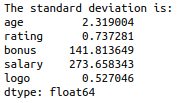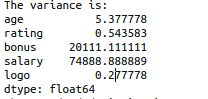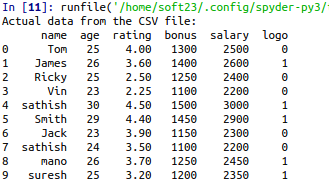• #5, First Floor, 4th Street Dr. Subbarayan Nagar Kodambakkam, Chennai-600 024 Landmark : Samiyar Madam
• pro@slogix.in
• +91- 81240 01111

### How to calculate the measure of dispersion for a data set using python?

###### Description

To calculate the measure of dispersion for a sample data set in python.

#### Range:

Difference between the largest and smallest values.

It takes largest and smallest value from the data set.

It uses only the data less than 6 in a data set.

#### Variance:

Variance measures how far a data set is spread out.

The average squared deviation of values from the mean.

#### Standard Deviation

It takes all data from the data set.

We can use standard deviation, if the data in a data set are more than 6.

Standard Deviation is the square root of variance.

###### Sample Code

#import pandas library

import pandas as pd

#load the data from CSV file

/Sathish/Pythonfiles/Employee.csv’)

#creating the Data Frame

df=pd.DataFrame(data)

print(“Actual data from the CSV file:”)

print(df)

#Calculate the variance

print(“The variance is:”)

print(df.var())

#calculate the standard deviation

print(“The standard deviation is:”)

print(df.std())

###### Screenshots